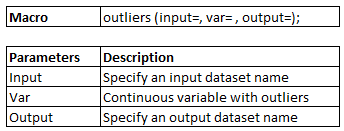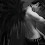# Identify and remove outliers with SAS

Detection of Outliers

If a value is higher than the 1.5 times of Interquartile Range (IQR) above the upper quartile (Q3), the value will be considered as mild-outlier. Similarly, if a value is lower than the 1.5 times of IQR below the lower quartile (Q1), the value will be considered as mild-outlier.

If a value is higher than the 3 times of Interquartile Range (IQR) above the upper quartile (Q3), the value will be considered as extreme-outlier. Similarly, if a value is lower than the 3 times of IQR below the lower quartile (Q1), the value will be considered as extreme-outlier.
IQR is interquartile range. It measures dispersion or variation. IQR = Q3 -Q1.
Lower limit of acceptable range = Q1 - 3* (Q3-Q1)
Upper limit of acceptable range = Q3 + 3* (Q3-Q1)

SAS Macro : Detect and Remove Outliers

The following macro calculates the lower and upper limit values of acceptable range and removes the observations that are outside this range. It works for multiple variables.SAS Macro : Detect and Remove Outliers

Updated - June17, 2016 - I have modified the code. The code works for removing outliers for multiple variables. For example, there are two continuous variables having extreme values. It will remove observations wherein extreme values exist. It can be same rows for both the variables or difference rows having extreme values for them.
%macro outliers(input=, var=, output= );

%let Q1=;
%let Q3=;
%let varL=;
%let varH=;

%let n=%sysfunc(countw(&var));
%do i= 1 %to &n;
%let val = %scan(&var,&i);
%let Q1 = &Q1 &val._P25;
%let Q3 = &Q3 &val._P75;
%let varL = &varL &val.L;
%let varH = &varH &val.H;
%end;

/* Calculate the quartiles and inter-quartile range using proc univariate */
proc means data=&input nway noprint;
var &var;
output out=temp P25= P75= / autoname;
run;

/* Extract the upper and lower limits into macro variables */
data temp;
set temp;
ID = 1;
array varb(&n) &Q1;
array varc(&n) &Q3;
array lower(&n) &varL;
array upper(&n) &varH;
do i = 1 to dim(varb);
lower(i) = varb(i) - 3 * (varc(i) - varb(i));
upper(i) = varc(i) + 3 * (varc(i) - varb(i));
end;
drop i _type_ _freq_;
run;

data temp1;
set &input;
ID = 1;
run;

data &output;
merge temp1 temp;
by ID;
array var(&n) &var;
array lower(&n) &varL;
array upper(&n) &varH;
do i = 1 to dim(var);
if not missing(var(i)) then do;
if var(i) >= lower(i) and var(i) <= upper(i);
end;
end;
drop &Q1 &Q3 &varL &varH ID i;
run;
%mend;

%outliers(input=tt, var= age weight height, output= outresult);
If you want to cap extreme values rather than removing them, you can check out the following link :
SAS Macro : Capping Outliers

Theory: Multiple ways to detect and solve problem of outliers
Related Posts
ShareDeepanshu founded ListenData with a simple objective - Make analytics easy to understand and follow. He has over 10 years of experience in data science. During his tenure, he worked with global clients in various domains like Banking, Insurance, Private Equity, Telecom and HR.

16 Responses to "Identify and remove outliers with SAS"
1.Thank you for this!

2.very good!

3.What does Range2 in the last line denote? Plz reply..only error is coming there..

4.Dude... That is how you have to run your function... If you are putting multiple variables than you have to give variable range & if only 1 variable then just give the variable name for which you are removing outliers.

5.hi Atul,

I've tried to modify your code and run it for multiple variables and it hasn't worked for me. Could you post an example of doing this with more than one variable?

I was wondering whether I need to introduce the scan(), to iterate through various vars, etc?

Thanks.. this is greatly appreciated. Makes me realize I dont understand SAS Macros.

6.Specifically what I did was create a macro variable in using the :varlist in proc sql, then I reference that &varlist when calling the outliers macro, i.e., %outliers(Input=test, Var = &varlist, Output= Outdata);

that doesn't work :(

1.Check out this version of macro. It works for multiple variables - http://www.listendata.com/2015/04/sas-macro-capping-outliers.html

2.Thanks, I'll take a look. You seem to have a lot more stuff on your blog. Great job :)

3.Cheers. I have modified the above code. Let me know if you face any issue(s). Thanks!

4.Hi Deepanshu,

I am loving your blog. What advice would you give someone who is trying to learn advanced SAS Macros fast? I've been using SAS for years, but I've never really had to write complex programs because there was no need to. Now I work with huge data sets, and I'm starting to see the need to catch up. I have Carpenter's Complete Guide to the SAS Macro Language, which I try to read. What else could I do? I plan to read through your tutorials also.

7.An issues I have with this macro is that if you want to run it for 50 variables then you have to list all of them here?

%outliers(input=tt, var= age weight height, output= outresult);

1.That works but it is not as elegant!!

Says someone who can't come up with anything close to this :)

2.An issue i have with your comment is you are posting as anonymous. I don't want to encourage readers to post comment as anonymous especially when they are seeking help. Thanks!

8.could you please explain how to remove outliers, I mean theoretical. How to identify and method I have understood,but how to remove I want to know.

9.It thought should always be 1.5 times IQR and not 3 times

10.As you have mentioned to calculate quartiles and interquartiles using proc univariate but you have used proc means. Please confirm if it univariate has to be used or proc means and do we have to modify something in the code?

Next → ← Prev
Looks like you are using an ad blocker!

To continue reading you need to turnoff adblocker and refresh the page. We rely on advertising to help fund our site. Please whitelist us if you enjoy our content.